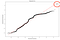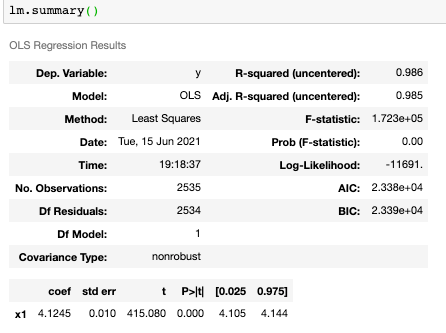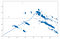# Outlier Study and Linear Regression Diagnosis using Python

As a data scientist working on sensor data of heating ventilating and air conditioning (HVAC) systems in buildings, we commonly apply anomaly detection statistical techniques or machine learning methods to detect and identify faults such as equipment failure or operational errors. Dealing with real-world sensor data has its own challenges.

One such use case is detecting faulty pumps used to facilitate the flow of chilled water to cool a building. This occurs when the increase or decrease in the power of a chilled water pump does not correspond to the increase or decrease of the chilled water flow rate of the pipes respectively.

Our approach was to train a regression model. Any deviation from a certain threshold of the subsequent predictions of new data, implies that an anomaly is detected. To ensure that the training data represents the optimal condition of the pump we needed to carefully explore and remove outliers.

For the longest time, I would identify outliers or irregularities in the data by flagging the points that deviate from the Interquartile Range (IQR). Interquartile Range (IQR) is defined as the difference between the third quartile and the first quartile (IQR = Q3 -Q1). Outliers, in this case, are defined as the observations that are below (Q1 − 1.5x IQR) or above (Q3 + 1.5x IQR). However, we soon realised we were removing far too many data points that were important for the regression. Thankfully, we came up with a more systematic approach in identifying outliers, by starting with the nature of the outliers we were faced with. We realised that the outliers we were faced with could be summarised in three main characteristics:

(a) Leverage: Whether a point is far away from the major cluster in the x-space. (b) Consistency: Whether a point is consistent in terms of fitting in the (x,y) space. (c) Influence: Whether a point highly affects the fitting of the model.

How do we identify such outliers? We use studentized deletion residuals as measures of consistency. The influence is measured via cook’s distance. For a measure on leverage, interestingly, we make use of the diagonals of the infamous OLS hat matrix: H = X * (transpose(X)*X)^(-1) * transpose(X). The hat value, h(i), are taken as leverage measures for the ith data point.

Below is the implementation in python, making use of a library called OLSInfluence from statsmodels. First, fit the regression model. The fitted regression object is then used to derive studentized residuals, leverage and the cook’s distance respectively.

The graph below is the Leverage v.s. studentized residuals plot. The y axis is the studentized residuals indicating if there are any outliers based on the alpha value (significance level). It shows points at the bottom right corner are high leverage data points. Generally there isn’t any issue with this regression fitting except for points 583 and 1758.

The informal assessment, usually by eye-balling Q-Q plots of studentized deletion residual, leverage h(i), and cook’s distance d(i) helps us make an assessment of the outliers and identify the indices of the points so that we can delete or keep them for our training. A more detailed explanation of the Q-Q plot can be read in the section below. The Q-Q plot of studentized residuals shows that indeed data point 583 is an outlier.Q-Q probability plot is heavy-tailed and shows a non-normal distribution with the outliers present.

Here is the code to retrieve the above Q-Q plot. Similar plots can be used for analysis of cook’s distance and leverage. Over here, we used probplot() from scipy.stats to generate the values.

However, a more formal and concrete assessment that can be used is to introduce the dummy variable, u, into our regression. The dummy variable will take on the value 1, for the ith unit (i.e. the data point we believe to be anomalous via our informal assessment) and 0 otherwise. The significance of this coefficient u in the linear predictor indicates and confirms that the ith point is indeed an outlier.

An outlier, unless its extremely influential, need not be excluded from the fitting of the model. But investigation is needed to find out the possibility of this influential data point.

Model Diagnosis using Python

One important aspect of modelling is to ensure that you are correctly diagnosing your model. The model could be experiencing systematic discrepancy and therefore severely hurting the performance of the prediction no matter how complex the model.

We are lucky that the linear regression generally performs well as the r-squared value is 0.985. However let’s continue to use this dataset and problem as a use case to evaluate if our data breaks any normality assumptions. We first fit the data, generate the regression function and its components such as the object itself, and also the summary table. In this case, we only have one predictor variable, the pump power. The response variable is the the flow speed.Left plot shows the regression function and the table on the right is the summary of the results of the fitted regression function.

Let us examine in detail the common discrepancies faced when assumptions of the linear regression are broken.

(a) The regression function is not linear. (b) The error terms do not have a constant variance. © The error terms are not independent. (d) The error terms are not normally distributed. (e) Some important predictors have been omitted from the model.

How do we identify which of these are the correct error diagnosis of the model? One Swiss army knife is by plotting residual plots to check for non-linearity, constant variance. If there are no discrepancies, the residuals would appear like random errors with mean zero, i.e. a NULL pattern. In any residual plot, the points would be scattered evenly within a horizontal band around zero. If a pattern exists, the model is a failure as it will not fit the regression function and we know if non-linearity or non-constant variance exists. Creating residuals plots against fitted values, and also residuals against predictor variables will be useful to find this out.

The seaborn package makes its convenient for us to plot residual plots as well as the smoother Lowess function just via a single line of code. In the code below, I constructed the studentized residuals vs. leverage plot. The response variable is the studentized residuals, the x-axis here is the leverage, as determined via the diagonal of the OLS hat matrix. In this case, there appears to be an upward, then a downward trend in the Lowess smoother, indicating a sign of heteroskedasticity.

Let’s also check if there is structure in the residuals relative to the fitted values. This plot is relatively straightforward to create. The plan here is to extract the residuals and fitted values from the fitted model, calculate a Lowess smoothed line through those points, then plot it out. In this case there may be a slight nonlinear structure in the residuals.Residual vs Fitted plot shows signs of nonlinearity, violating the null pattern.

Another Swiss army knife is the Q-Q plot or the normal probability plot, which we have touched on in the above section. The Q-Q plot helps us identify normality conditions of the predictor or the error terms. The NULL pattern of the Q-Q plot is that the points should fall alone in a straight line. If it’s a convex curve, the distribution is non-symmetric and skewed and is better explained via a gamma distribution. Symmetric but heavy-tailed distributions also violate the NULL pattern of the Q-Q plot, meaning that the distribution of the data is not normal and its better to conduct some transformations before fitting the regression model. More details and excellent explanations on the different types of Q-Q plots can be found in this blog post that I found: https://towardsdatascience.com/q-q-plots-explained-5aa8495426c0

In summary for model diagnostics, firstly, check for non-linearity. There are a few residual plots that can be used to check this condition. Firstly, plot a residual plot against fitted values, then a residual plot against each predictor variables. Additionally, when you have more than one predictor variable, a scatter plot of response against predictor variables can help in identifying the relationship with the response variable.

Secondly, when checking for homogeneity, residual plots against fitted values and residual plot against predictor variables will suffice. We need to see that the variance of residuals is a constant, i.e. the points appear random. This gives us the confidence that the error terms are independently distributed.

Lastly, but certainly not the least, checking for normality. The Q-Q plot is really efficient in identifying any skew in the distribution of the response variable and can help us decide if transformations of the x, y variables are required. Normality is an important assumption to successfully fit a model.

I hope this is a useful summary for data scientist who are busy fitting linear regression models but unable to identify and remedy abnormalities in the real world data set. Do note that it is much difficult to provide such diagnosis when using Sklearn libraries for linear regression. Hence, I highly recommend using statsmodels or scipy.stats for linear regression type of problems in python.

Hope you enjoyed my post! Feel free to learn and share the findings and feel free to add me on LinkedIn: https://www.linkedin.com/in/esther-dawes-9b462343/ and follow some of my work on github where I try my best to post interesting projects every now and then: https://github.com/technologic27.

## CodeX

Everything connected with Tech & Code

Everything connected with Tech & Code

Written by

## Esther Dawes

An aspiring data scientist, with a passion for data-driven decision-making.